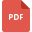# mathematics grade 5 united states tutorialsRoman Numerals: Explained with Sample Problems
Mathematics, Grade 5, United States, Roman NumeralsReciprocals of Fractions
Fraction, Grade 5, United States, MathematicsAverages, Means, Medians and Modes
Statistics, Grade 5, United States, MathematicsProportions: True/False Proportions, Application Problems
Ratio and Proportion, Grade 5, United States, MathematicsEstimating Sum and Difference of Decimal NumbersLine of Symmetry and Rotation of Figures
Symmetry, Grade 5, United States, MathematicsFraction in Highest Terms
Fraction, Grade 5, United States, MathematicsMultiplying by 10, 100, and 1,000
Multiplication, Grade 5, United States, MathematicsPolygons: Definition and Identification
Mathematics, Grade 5, United States, PolygonsDetailed Explanation of Mixed Numbers
Mathematics, Grade 5, United States, Number SystemComparing and Ordering Fractions Worksheet
Fraction, Grade 5, United States, MathematicsDetailed Explanation of Place Value
Place Value, Grade 5, United States, MathematicsSolved Worksheet on Estimating Quotients
Division, Grade 5, United States, MathematicsMeasuring Length and Distances: Relating Customary Units of Length
Measurement, Grade 5, United States, MathematicsProper and Improper Fractions
Fraction, Grade 5, United States, MathematicsAdding Decimals upto 3 Decimal PlacesIdentifying Angles: Acute Angle, Right Angle and Obtuse Angle
Right Triangle, Grade 5, United States, Lines and Angles, Mathematics, GeometryDetailed Explanation of Greatest Common Factor
Mathematics, Grade 5, United States, HCFShort Division Method: Explained
Division, Grade 5, United States, MathematicsIntroductions to Decimal Numbers
Decimals and Multiplication, Grade 5, United States, MathematicsDividing Fractions by another Fractions
Fraction, Grade 5, United States, MathematicsProperties of Multiplication
Multiplication, Grade 5, United States, MathematicsAddition, Subtraction, Multiplication, Division of Fractions
Fraction, Grade 5, United States, MathematicsIntroduction to Probability with Sample Questions
Probability, Grade 5, United States, MathematicsCollecting, Organizing and Working with Data
Data, Grade 5, United States, Mathematics